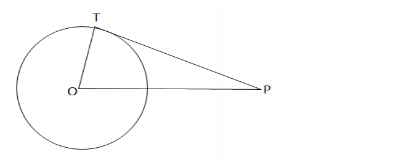# A point P is 25 cm away from the centre of a circle and the length of tangent drawn from P to the circle is 24 cm.Question:

A point P is 25 cm away from the centre of a circle and the length of tangent drawn from to the circle is 24 cm. Find the radius of the circle.

Solution:Draw a circle and let $P$ be a point such that $O P=25 \mathrm{~cm}$.

Let $T P$ be the tangent, so that $T P=24 \mathrm{~cm}$

Join $O T$, where $O T$ is radius.

Now, tangent drawn from an external point is perpendicular to the radius

at the point of contact.

$\therefore O T \perp P T$

In the right $\triangle O T P$, we have:

$O P^{2}=O T^{2}+T P^{2} \quad[$ By Pythagoras' theorem: $]$

$O T^{2}=\sqrt{O P^{2}-T P^{2}}$

$=\sqrt{25^{2}-24^{2}}$

$=\sqrt{625-576}$

$=\sqrt{49}$

$=7 \mathrm{~cm}$

$\therefore$ The length of the radius is $7 \mathrm{~cm}$.